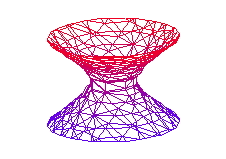Scientific WorkPlace and Scientific Notebook use the MuPAD 2.0 kernel, which is the same as in the full version of MuPAD 2.0. A new interface to the kernel to make MuPAD easy to use with Scientific WorkPlace and Scientific Notebook. In addition, the system accepts input and create output using natural mathematical notation, the basis for our scientific word processors. Performing computations in Scientific WorkPlace and Scientific Notebook is even easier than using the system directly.

Computational Functions. Scientific WorkPlace and Scientific Notebook provide a wide range of the graphic, numeric, and symbolic computational functions available with MuPAD. The programs provide ample functionality for both simple and sophisticated mathematical computations involving calculus, PDE, ODE, matrix manipulations, statistics, linear algebra, and 2D and 3D plots. Also, you can access additional functions available to the systems—even if they don’t appear as items on the Compute menu—with the Define MuPAD Name menu items.

User-defined Functions. With MuPAD, you can create user-defined functions (.mu files) with an ASCII editor, even if you don’t have access to a full MuPAD installation. The files are easy to manipulate and are powerful tools for users interested in programming. Working in a Scientific WorkPlace or Scientific Notebook document, you call the function with the Define MuPAD Name command.

Available Functions. While Scientific WorkPlace and Scientific Notebook provide many functions available with MuPAD, not all capabilities are included. Programming packages, certain plot types and options (especially animated plots), and manipulation of the position of highlights and shadows in 3D plots aren’t available. Scientific Notebook and Scientific Workplace do not have 3D implicit plotting. Additionally, some limitations exist regarding the placement of text on plots and the use of different types of plots on the same graph. Iteration and condition commands (such as if, elif, else, fi, for, while, do, and od) aren’t available.

Examples

You can use MuPAD to solve these kinds of problems in Scientific WorkPlace and Scientific Notebook:

Arithmetic

Evaluate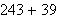Factor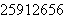Evaluate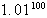Evaluate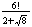SimplifyFactorEvaluate NumericallyAlgebra

Expand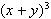Factor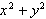Solve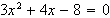Calculus

Evaluate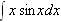Evaluate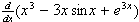Evaluate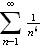Linear Algebra

Evaluate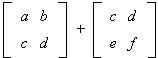Evaluate the Determinant of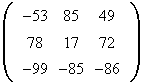Statistics

Determine the Mean of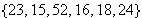Determine the Standard Deviation ofPlots

You can create plots like these with Scientific WorkPlace and Scientific Notebook:

Here is a sample of some graphs you can create with Scientific WorkPlace and Scientific Notebook:

Plot 2D + Rectangular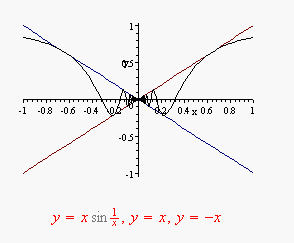Piecewise-Defined Function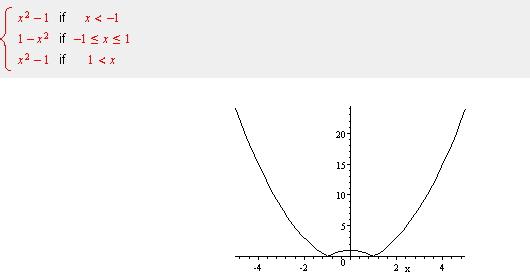Bar Charts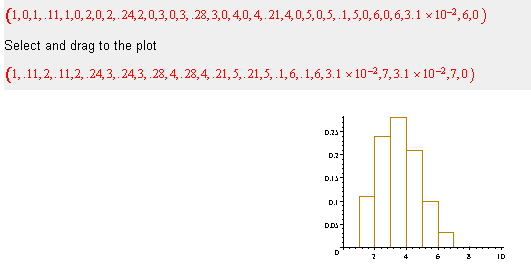Grids (you can create a grid using point plots)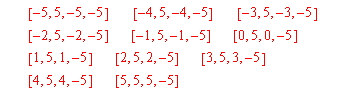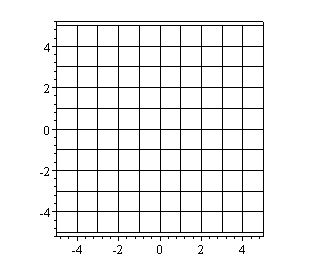3D Graphs